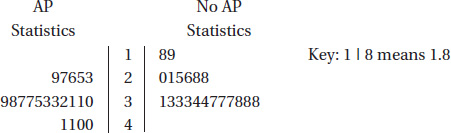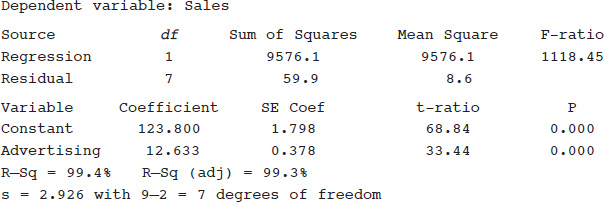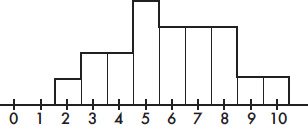# AP Statistics Multiple-Choice Practice Test 26

### Test Information10 questions23 minutes

1. Two random samples of students are chosen, one from those taking an AP Statistics class and one from those not. The following back-to-back stemplots compare the GPAs.Which of the following is true about the ranges and standard deviations?

2. In a group of 10 scores, the largest score is increased by 40 points. What will happen to the mean?

3. Suppose X and Y are random variables with μx = 32, σx = 5, μy = 44, and σy = 12. Given that X and Y are independent, what are the mean and standard deviation of the random variable X + Y?

4. Suppose you toss a fair die three times and it comes up an even number each time. Which of the following is a true statement?

5. A pharmaceutical company is interested in the association between advertising expenditures and sales for various over-the-counter products. A sales associate collects data on nine products, looking at sales (in \$1000) versus advertising expenditures (in \$1000). The results of the regression analysis are shown below.Which of the following gives a 90% confidence interval for the slope of the regression line?

6. Suppose you wish to compare the AP Statistics exam results for the male and female students taking AP Statistics at your high school. Which is the most appropriate technique for gathering the needed data?

7. Jonathan obtained a score of 80 on a statistics exam, placing him at the 90th percentile. Suppose five points are added to everyone's score. Jonathan's new score will be at the

8. To study the effect of music on piecework output at a clothing manufacturer, two experimental treatments are planned: day-long classical music for one group versus day-long light rock music for another. Which one of the following groups would serve best as a control for this study?

9. Suppose H0: p = 0.6, and the power of the test for Ha: p = 0.7 is 0.8. Which of the following is a valid conclusion?

10. Following is a histogram of the numbers of ties owned by bank executives.Which of the following is a correct statement ?